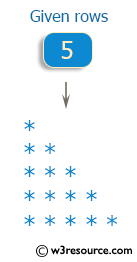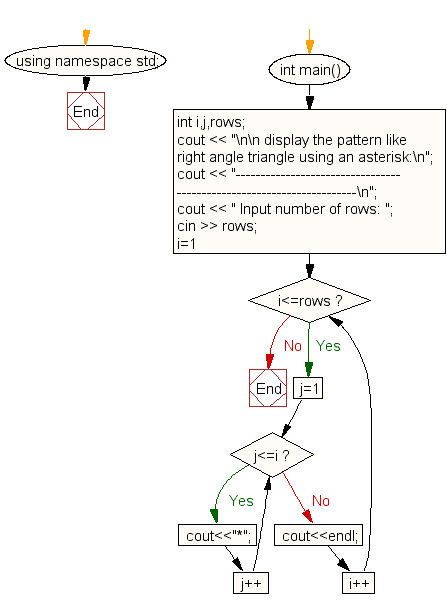﻿ C++ : Pattern like right angle triangle using an asterisk

# C++ Exercises: Display the pattern like right angle triangle using an asterisk

## C++ For Loop: Exercise-35 with Solution

Write a program in C++ to display a pattern like a right angle triangle using an asterisk.

Visual Presentation:Sample Solution:-

C++ Code :

``````#include <iostream> // Include the input/output stream library
using namespace std; // Using standard namespace

int main() // Main function where the execution of the program starts
{
int i, j, rows; // Declare integer variables i, j, and rows

// Display message asking for input
cout << "\n\n display the pattern like right angle triangle using an asterisk:\n";
cout << "---------------------------------------------------------------------\n";
cout << " Input number of rows: ";
cin >> rows; // Read input for the number of rows from the user

// Loop to print the right angle triangle pattern with asterisks
for (i = 1; i <= rows; i++) // Loop for the number of rows
{
for (j = 1; j <= i; j++) // Nested loop for printing asterisks in each row
{
cout << "*"; // Print an asterisk
}
cout << endl; // Move to the next line after each row is printed
}
}
``````

Sample Output:

``` display the pattern like right angle triangle using an asterisk:
---------------------------------------------------------------------
Input number of rows: 5
*
**
***
****
*****
```

Flowchart:C++ Code Editor: